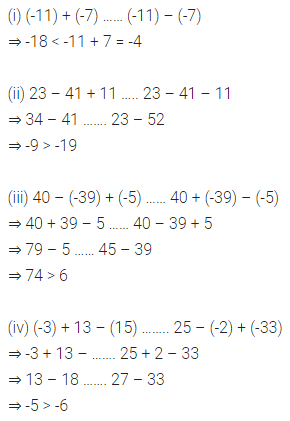# ML Aggarwal Class 7 Solutions for ICSE Maths Chapter 1 Integers Ex 1.1

## ML Aggarwal Class 7 Solutions for ICSE Maths Chapter 1 Integers Ex 1.1

Question 1.
Some integers are marked on the following number line:(i) Write these integers in ascending order.
(ii) Write these integers in descending order.
(iii) Few dots have been marked on the above number line. Write an appropriate integer at each dot.
Solution: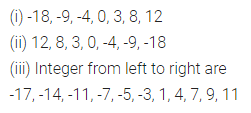Question 2.
A number line representing integers is given below: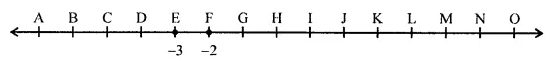The integers -3 and -2 are marked by points E and F respectively. Which integers are marked by points B, D, H, J, M and O?
Solution:Question 3.
Arrange 7, -5, 4, 0 and -4 in ascending order and mark them on a number line to check your answer.
Solution: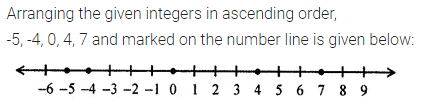Question 4.
In a quiz, positive marks are given for correct answers and negative marks are given for incorrect answers. If Rohit’s scores in five successive rounds were 15, -3, -7, 12 and 8, what was his total at the end?
Solution: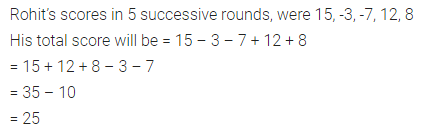Question 5.
Ruchi deposited ₹ 4370 in her account on Monday and then withdrew ₹ 2875 on Tuesday. Next day she deposited ₹ 1550. What was her balance on Thursday?
Solution: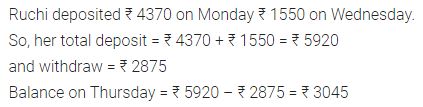Question 6.
Ravi goes 28 km towards east from a point P to the point Q. From Q, he moves 37 km towards the west along the same road. If the distance towards east is represented by a positive integer then, how will you represent the distance travelled towards the west? By which integer will you represent his final position from P?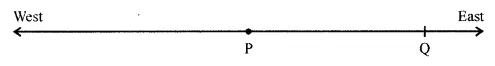Solution: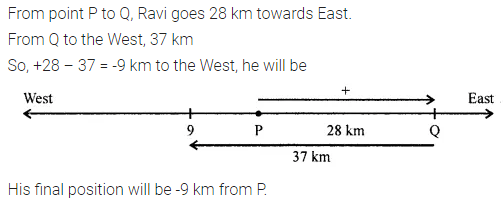Question 7.
Fill in the blank spaces by integers of the given magic square so that the sum of the integers in each row, each column and each diagonal is -6.Solution: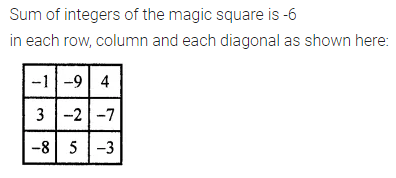Question 8.
Evaluate the following:
(i) |-13| – |9|
(ii) |13 – 5| – |-9|
(iii) |35 – 21| – |8 – 3|
Solution: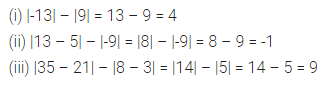Question 9.
Arrange the following integers in ascending order:
-39, 35, -102, 0, -51, -5, -6, 7
Solution: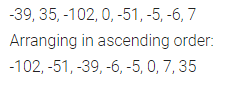Question 10.
Arrange the following integers in descending order:
-31, 139, -203, -97, 0, 4208
Solution:Question 11.
State whether each of the following statement is true or false:
(i) 0 is the successor of -1 in integers
(ii) 0 has no predecessor in integers
(iii) -2 is the predecessor of -1
(iv) 0 is greater than every negative integer.
Solution:Question 12.
Use the sign >, < or = in the box to make the following statements true:
(i) (-11) + (-7) ……….. (-11) – (-7)
(ii) 23 – 41 + 11 ……… 23 – 41 – 11
(iii) 40 – (-39) + (-5) …….. 40 + (-39) – (-5)
(iv) (-3) + 13 – (15) ……. 25 – (-2) + (-33)
Solution: